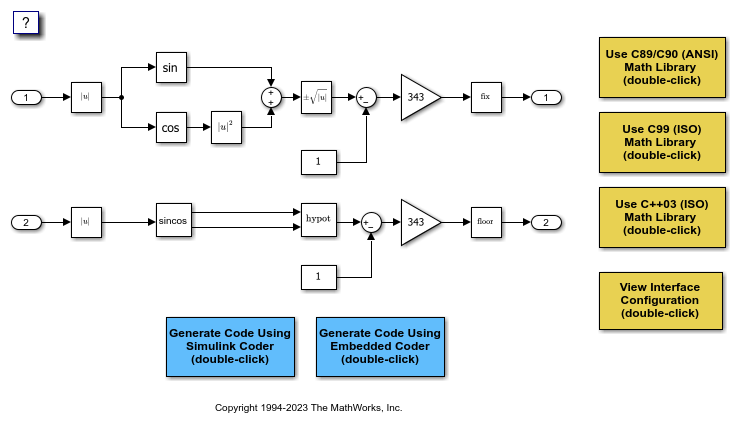# Configure Standard Math Library for Target System

Specify standard library extensions that the code generator uses for math operations. When you generate code for a new model or with a new configuration set object, the code generator uses the ISO®/IEC 9899:1999 C (C99 (ISO)) library by default. For preexisting models and configuration set objects, the code generator uses the library specified by the Standard math library parameter.

If your compiler supports the ISO®/IEC 9899:1990 (C89/C90 (ANSI)), ISO/IEC 14882:2003(C++03 (ISO) or ISO/IEC 14882:2011(C++11 (ISO)) math library extensions, you can change the standard math library setting. The C++03 (ISO) or C++11 (ISO) library is an option when you select C++ for the programming language.

The C99 library leverages the performance that a compiler offers over standard ANSI C. When using the C99 library, the code generator produces calls to ISO C functions when possible. For example, the generated code calls the function `sqrtf()`, which operates on single-precision data, instead of `sqrt()`.

To change the library setting, use the Configuration Parameters>Standard math library parameter. The command-line equivalent is `TargetLangStandard`.

### Generate and Inspect ANSI C Code

1. Open the example model `rtwdemo_clibsup`.2. Generate code.

```### Starting build procedure for: rtwdemo_clibsup ### Successful completion of code generation for: rtwdemo_clibsup Build Summary Top model targets built: Model Action Rebuild Reason ==================================================================================== rtwdemo_clibsup Code generated. Code generation information file does not exist. 1 of 1 models built (0 models already up to date) Build duration: 0h 0m 9.1844s ```

3. Examine the code in the generated file `rtwdemo_clibsup.c`. Note that the code calls the `sqrt` function.

``` if (rtb_Abs2 < 0.0F) { rtb_Abs2 = -(real32_T)sqrt((real32_T)fabs(rtb_Abs2)); } else { rtb_Abs2 = (real32_T)sqrt(rtb_Abs2); } /* Gain: '<Root>/Gain1' incorporates: * Constant: '<Root>/Constant' * Sqrt: '<Root>/get_hypot1' * Sum: '<Root>/Sum' * * About '<Root>/get_hypot1': * Operator: signedSqrt */ rtb_Abs2 = (rtb_Abs2 - 1.0F) * 343.0F; /* Rounding: '<Root>/fix1' */ if (rtb_Abs2 < 0.0F) { /* Outport: '<Root>/Out1' */ rtwdemo_clibsup_Y.Out1 = (real32_T)ceil(rtb_Abs2); } else { /* Outport: '<Root>/Out1' */ rtwdemo_clibsup_Y.Out1 = (real32_T)floor(rtb_Abs2); } /* End of Rounding: '<Root>/fix1' */ /* Abs: '<Root>/Abs2' incorporates: * Inport: '<Root>/In2' */ rtb_Abs2 = (real32_T)fabs(rtwdemo_clibsup_U.In2); /* Outport: '<Root>/Out2' incorporates: * Constant: '<Root>/Constant1' * Gain: '<Root>/Gain2' * Math: '<Root>/get_hypot2' * Rounding: '<Root>/fix2' * Sum: '<Root>/Sum2' * Trigonometry: '<S1>/cos' * Trigonometry: '<S1>/sin' */ rtwdemo_clibsup_Y.Out2 = (real32_T)floor((rt_hypotf_snf((real32_T)sin(rtb_Abs2), (real32_T)cos(rtb_Abs2)) - 1.0F) * 343.0F); } /* Model initialize function */ void rtwdemo_clibsup_initialize(void) { /* Registration code */ /* initialize non-finites */ rt_InitInfAndNaN(sizeof(real_T)); /* initialize error status */ rtmSetErrorStatus(rtwdemo_clibsup_M, (NULL)); /* external inputs */ (void)memset(&rtwdemo_clibsup_U, 0, sizeof(ExtU_rtwdemo_clibsup_T)); /* external outputs */ (void)memset(&rtwdemo_clibsup_Y, 0, sizeof(ExtY_rtwdemo_clibsup_T)); } /* Model terminate function */ void rtwdemo_clibsup_terminate(void) { /* (no terminate code required) */ } ```

### Generate and Inspect ISO C Code

1. Change the setting of Standard math library to `C99 (ISO)`. Alternatively, at the command line, set `TargetLangStandard` to `C99 (ISO)`.

2. Regenerate the code.

```### Starting build procedure for: rtwdemo_clibsup ### Successful completion of code generation for: rtwdemo_clibsup Build Summary Top model targets built: Model Action Rebuild Reason ==================================================================================== rtwdemo_clibsup Code generated. Code generation information file does not exist. 1 of 1 models built (0 models already up to date) Build duration: 0h 0m 8.0284s ```

3. Reexamine the code in the generated file `rtwdemo_clibsup.c`. Now the generated code calls the function `sqrtf` instead of `sqrt`.

``` if (rtb_Abs2 < 0.0F) { rtb_Abs2 = -sqrtf(fabsf(rtb_Abs2)); } else { rtb_Abs2 = sqrtf(rtb_Abs2); } /* Outport: '<Root>/Out1' incorporates: * Constant: '<Root>/Constant' * Gain: '<Root>/Gain1' * Rounding: '<Root>/fix1' * Sqrt: '<Root>/get_hypot1' * Sum: '<Root>/Sum' * * About '<Root>/get_hypot1': * Operator: signedSqrt */ rtwdemo_clibsup_Y.Out1 = truncf((rtb_Abs2 - 1.0F) * 343.0F); /* Abs: '<Root>/Abs2' incorporates: * Inport: '<Root>/In2' */ rtb_Abs2 = fabsf(rtwdemo_clibsup_U.In2); /* Outport: '<Root>/Out2' incorporates: * Constant: '<Root>/Constant1' * Gain: '<Root>/Gain2' * Math: '<Root>/get_hypot2' * Rounding: '<Root>/fix2' * Sum: '<Root>/Sum2' * Trigonometry: '<S1>/cos' * Trigonometry: '<S1>/sin' */ rtwdemo_clibsup_Y.Out2 = floorf((rt_hypotf_snf(sinf(rtb_Abs2), cosf(rtb_Abs2)) - 1.0F) * 343.0F); } /* Model initialize function */ void rtwdemo_clibsup_initialize(void) { /* Registration code */ /* initialize non-finites */ rt_InitInfAndNaN(sizeof(real_T)); /* initialize error status */ rtmSetErrorStatus(rtwdemo_clibsup_M, (NULL)); /* external inputs */ (void)memset(&rtwdemo_clibsup_U, 0, sizeof(ExtU_rtwdemo_clibsup_T)); /* external outputs */ (void)memset(&rtwdemo_clibsup_Y, 0, sizeof(ExtY_rtwdemo_clibsup_T)); } /* Model terminate function */ void rtwdemo_clibsup_terminate(void) { /* (no terminate code required) */ } ```